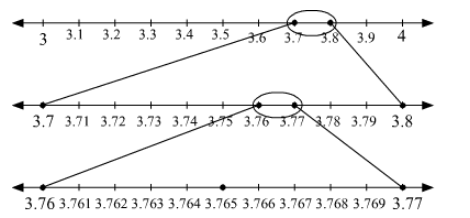# Visualize the representation of 3.765 on

Question:

Visualize the representation of 3.765 on the number line using successive magnification.

Solution:

3 < 3.765 < 4
Divide the gap between 3 and 4 on the number line into 10 equal parts.
Now, 3.7 < 3.765 < 3.8
In order to locate the point 3.765 on the number line, divide the gap between 3.7 and 3.8 into 10 equal parts.
Further, 3.76 < 3.765 < 3.77
So, to locate the point 3.765 on the number line, again divide the gap between 3.76 and 3.77 into 10 equal parts.
Now, the number 3.765 can be located on the number line. This can be shown as follows:Here, the marked point represents the point 3.765 on the number line.